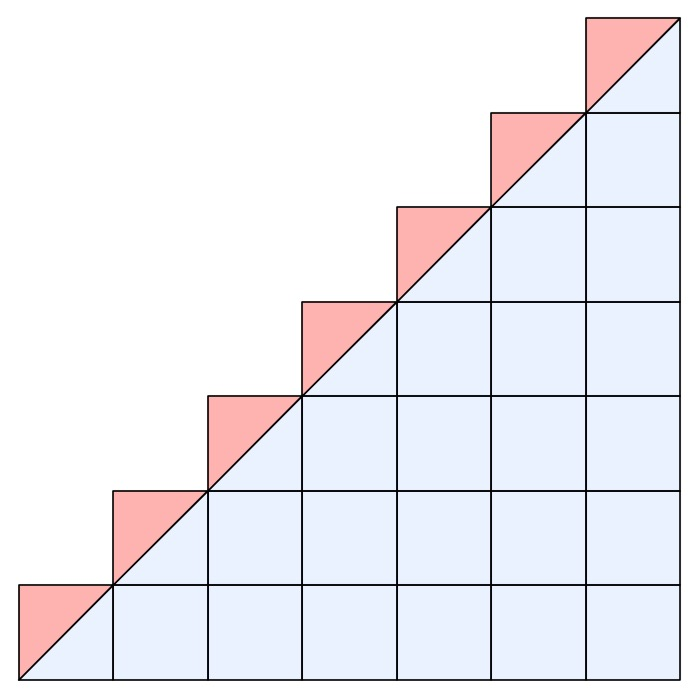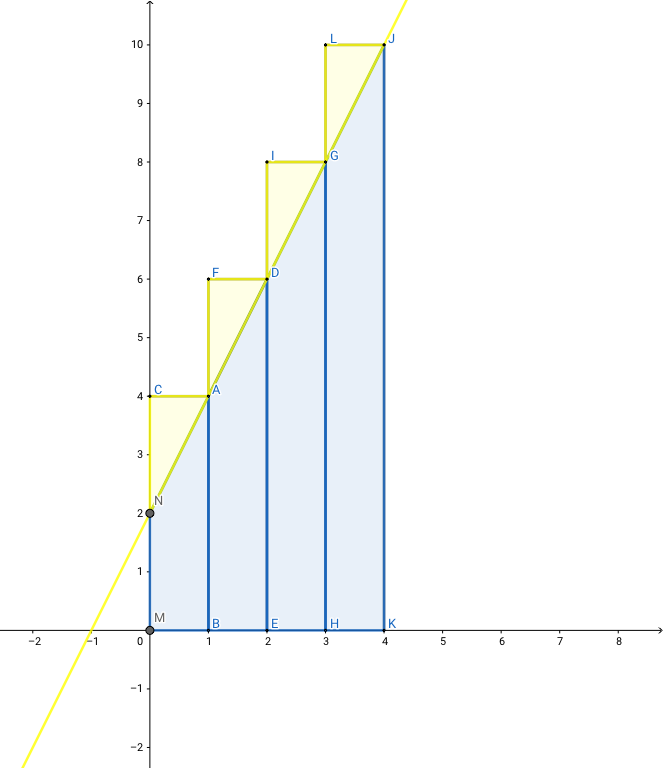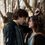# Something Interesting in Arithmetic Sequences

## $1+2+3+\cdots +n$

I believe you are familiar with this formula. The German mathematician Gauss is said to have studied the formula at the age of three. To this day, there are many explanations. The most widely used method, which Gauss used in those days, was to add the first and last terms, the second and the penultimate.

For the arithmetic progression $1,2,3,\cdots ,n$, there are $\displaystyle \sum_{i=1}^n i=\dfrac{n^2}{2}+\dfrac{n}{2}$ For the arithmetic progression $a_1,a_2,a_3\cdots a_n$, if $a_n-a_{n-1}=d$, there are $a_n=a_1+(n-1)d$ $d=\dfrac{a_m-a_n}{m-n}$ $a_1+a_n=a_2+a_{n-1}=\cdots =a_k+a_{n-k+1}$ $\displaystyle \sum_{i=1}^n a_i=\dfrac{(a_1+a_n)\times n}{2}=a_1\times n+\dfrac{n(n-1)\times d}{2}$

For example:

$(1)$ Find $1+2+3+\cdots +50$. $(2)$ Find $1+2+3+\cdots +100$

\begin{aligned} (1) S &=1+2+3+\cdots +50 \\ &=(1+50)+(2+49)+(3+48)+\cdots \\ &=51\times \dfrac{50}{2} \\ &=1275 \end{aligned}

\begin{aligned} (2) S &=1+2+3+\cdots +100 \\ &=(1+100)+(2+99)+(3+98)+\cdots \\ &=101\times \dfrac{100}{2} \\ &=5050 \end{aligned}

From the above example, we can get $1+2+3+\cdots +n=\dfrac{n^2}{2}+\dfrac{n}{2}$. But this explanation is flawed. When the number of terms is an odd number, there is always a number in the middle that cannot be paired with other Numbers. If I had to figure out the middle number, it would have been too much trouble. Like this example. We can do it the way we did it above, and there are many ways to do it. But let's think about it another way. We can eliminate this trouble by constructing two sequences ${1,2,3\cdots 2n+1}$ and ${2n+1,2n,2n-1\cdots 1}$, and adding the $n^{th}$ term of the first sequence to the $n^{th}$ term of the second sequence.

Find $1+2+3+\cdots +101$

\begin{aligned} S &=1+2+3+\cdots +101 \\ &=\dfrac{1}{2}\times [(1+2+3+\cdots +101)+(101+100+99+\cdots +1)] \\ &=\dfrac{1}{2}\times [(1+101)+(2+100)+(3+99)+\cdots] \\ &=\dfrac{1}{2}\times (1+101)\times 101 \\ &=5151 \end{aligned}

The following picture also proves our derivation. This image comes from Basic Mathematics. In the picture, $S=1+2+3+\cdots +n=\dfrac{n^2}{2}+\dfrac{n}{2}$. We can see that the equation of line in the figure is $y=x$. It also shows that there is a close relationship between arithmetic progression and the Linear function $y=kx+b$.## $a_n=a_1+(n-1)d$ and $y=kx+b$

When the teacher talks about this, someone always asks this question. In fact, this problem can be solved by the general formula of arithmetic progression. For any arithmetic sequence $a_n$,there are

$a_1+a_n=a_2+a_{n-1}=\cdots =a_k+a_{n-k+1}$
We combined Linear Function with arithmetic sequence, and set $a_1=k+b$, $a_n=kn+b$. So we can also understand $\displaystyle\int_0^n (kx+b) \mathrm{d}x+\dfrac{1}{2}kn=\displaystyle\sum_{i=1}^n a_i$. This needs to do a lot of tedious promotion, here I will not write.\begin{aligned} \int_0^n (kx+b) \mathrm{d}x+\dfrac{1}{2}kn &=\left.\int (kx+b) \mathrm{d}x\right|_0^n+\dfrac{1}{2}kn \\ &=\left.\dfrac{1}{2}kx^2+bx+\mathrm {C}\right|_0^n+\dfrac{1}{2}kn \\ &=\dfrac{1}{2}kn^2+bn+\dfrac{1}{2}kn \\ &=\dfrac{n}{2}(kn+2b+k) \\ &=\dfrac{n}{2}[(kn+b)+(k+b)] \\ &=\dfrac{(a_1+a_n)n}{2} \end{aligned}

## The sum of arithmetic sequence

The above example is an arithmetic sequence with a tolerance equal to one. For arithmetic progression

$a_1,a_2,a_3\cdots a_n$

We can use the above idea to solve the problem. First, we tried to use algebraic methods.

$\displaystyle \sum_{i=1}^n a_i=\dfrac{1}{2}[(a_1+a_n)+(a_2+a_{n-1})+\cdots]=\dfrac{(a_1+a_n)\times n}{2}$

If we substitute $a_n$, we can get:

$\displaystyle \sum_{i=1}^n a_i=\dfrac{[2a_1+(n-1)d]\times n}{2}=a_1\times n+\dfrac{n(n-1)\times d}{2}$

There is another way to do this. Thanks to my friend Alex Dixon here, I have modified his method slightly, as follows:

\begin{aligned} \because a_n &=a_1+(n-1)d \\ \therefore \displaystyle \sum_{i=1}^n a_i &=[a_1+(1-1)d]+[a_1+(2-1)d]+[a_1+(3-1)d]+\cdots +[a_1+(n-1)d] \\ &=a_1\times n+[d+2d+3d+\cdots +(n-1)d] \\ &=a_1\times n+[1+2+3+\cdots +(n-1)]d \\ &=a_1\times n+\dfrac{n(n-1)\times d}{2} \end{aligned}

And once again, we can prove it with the pattern above. But it needs to be changed a little bit. I'm sorry that I can't submit pictures here. Those who are interested can draw a picture by yourselves. And you'll see that it becomes a complete trapezoid. And using mathematical induction, of course. Like this:

When $n=1$, $S_1=a_1$, $S_2=a_1\times 1+\dfrac{1\times (1-1)\times d}{2}=a_1$. There's $S_1=S_2$, so it works.

If $n=k$, there's $\displaystyle \sum_{i=1}^k a_i=a_1\times k+\dfrac{kd(k-1)}{2}$.

When $n=k+1$, we can get

\begin{aligned} \displaystyle \sum_{i=1}^{k+1} a_i &=a_1\times k+\dfrac{k(k-1)\times d}{2}+a_1+kd \\ &=a_1\times (k+1)+\dfrac{k^2d-kd}{2}+kd \\ &=a_1\times (k+1)+\dfrac{k^2d+kd}{2} \\ &=a_1\times (k+1)+\dfrac{(k+1)[(k+1)-1]\times d}{2} \end{aligned}Note by Edward Christian
1 year, 8 months ago

This discussion board is a place to discuss our Daily Challenges and the math and science related to those challenges. Explanations are more than just a solution — they should explain the steps and thinking strategies that you used to obtain the solution. Comments should further the discussion of math and science.

When posting on Brilliant:

• Use the emojis to react to an explanation, whether you're congratulating a job well done , or just really confused .
• Ask specific questions about the challenge or the steps in somebody's explanation. Well-posed questions can add a lot to the discussion, but posting "I don't understand!" doesn't help anyone.
• Try to contribute something new to the discussion, whether it is an extension, generalization or other idea related to the challenge.
• Stay on topic — we're all here to learn more about math and science, not to hear about your favorite get-rich-quick scheme or current world events.

MarkdownAppears as
*italics* or _italics_ italics
**bold** or __bold__ bold
- bulleted- list
• bulleted
• list
1. numbered2. list
1. numbered
2. list
Note: you must add a full line of space before and after lists for them to show up correctly
paragraph 1paragraph 2

paragraph 1

paragraph 2

[example link](https://brilliant.org)example link
> This is a quote
This is a quote
    # I indented these lines
# 4 spaces, and now they show
# up as a code block.

print "hello world"
# I indented these lines
# 4 spaces, and now they show
# up as a code block.

print "hello world"
MathAppears as
Remember to wrap math in $$ ... $$ or $ ... $ to ensure proper formatting.
2 \times 3 $2 \times 3$
2^{34} $2^{34}$
a_{i-1} $a_{i-1}$
\frac{2}{3} $\frac{2}{3}$
\sqrt{2} $\sqrt{2}$
\sum_{i=1}^3 $\sum_{i=1}^3$
\sin \theta $\sin \theta$
\boxed{123} $\boxed{123}$

## Comments

Sort by:

Top Newest

I am familiar with the theorem but have never viewed it like this.

- 11 months ago

Log in to reply

How can you be such a great mathematician you’re only 13!

- 11 months ago

Log in to reply

You're welcome Edward

- 1 year, 7 months ago

Log in to reply

Of course, you can

- 1 year, 8 months ago

Log in to reply

Check this out:

For an arithmetic sequence, an+b the equation can be written as x1 + x2 + x3 +…+ xn = (a+b) + (2a + b) + (3a + b) +…+ (an+b) => (a + 2a + 3a +…+ an) + (b + b + b +… to n terms) = a[1 + 2 + 3 +…+n] + nb = [a(n(n+1))/2] + nb = [an^2+ an]/2+ nb = n/2 [an+a+2b] = n/2 [an+a+b+b] = n/2 [(a+b)+(an+b)] In this sequence, (a+b) = x1 , and (an+b) = xn So, this equation can be written in terms of first and last term as, x1 + x2 + x3 +…+ xn = n/2 [x1+ xn]

- 1 year, 8 months ago

Log in to reply

Emm...I think this is a great idea.CanI add it to my note?

- 1 year, 8 months ago

Log in to reply

We can derive more equations from the above. This equation can rewritten using the first and last terms of the sequence. You know, we can use different forms of the same equation in different problems. That's the interesting fact about mathematics. It's interesting, isn't it?

- 1 year, 8 months ago

Log in to reply

Yes, Sir. The above problem is actually a special arithmetic sequence. And if we keep going, we're going to get a lot more interesting results. But I have to admit that, first of all, I wrote this NOTE in a hurry. Second, this NOTE is mainly intended to state an idea, not a result. Thirdly, I am only a middle school student with limited math skills. These are the only things I can say in a rigorous and methodical way. Although I have a lot of ideas, I am not very mature enough to write them here. If you have a better idea, please let me know.

- 1 year, 8 months ago

Log in to reply

×

Problem Loading...

Note Loading...

Set Loading...